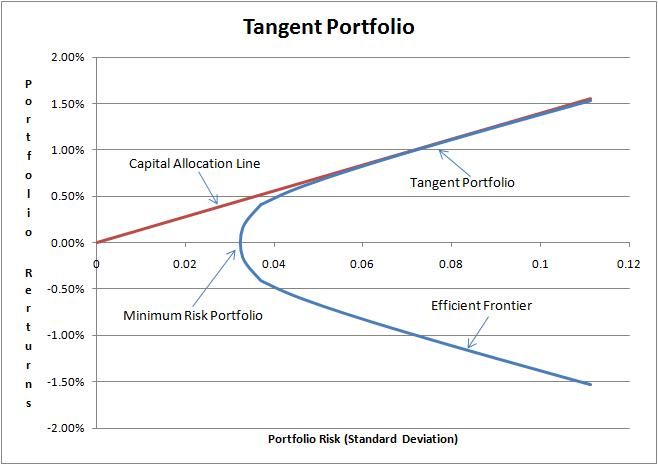# Markowitz Optimal Portfolio

Harry Markowitz first introduced in 1952 his Modern Portfolio Theory. William Sharpe formalized the Capital Asset Pricing Model (CAPM) that completes the original work of Markowitz. The basic concept is to try to optimize a portfolio of assets maximizing the returns and minimizing the risks of this portfolio. As a basic measure of risk, Markowitz assumed the standard deviation of the returns. The theory says that the risk of a diversified stock portfolio (or asset classes portfolio) is less than the risk of owning one individual stock (or asset class). The real problem is then determining the proportion of each stock or asset class in the portfolio so to maximize return and minimize risk. A portfolio that contains this balanced mix will perform well regardless of stock market conditions.

The theory has been criticized because of the following basic wrong assumptions:

• Returns follow a Gaussian distribution.
• The correlation between assets is constant (not varying with time).
• The Market is an efficient environment and the Efficient Market Hypothesis is true.

Despite these well-known issues, the Markowitz theory is still widely used in finance and the concepts behind it broadly influenced the industry. How are we supposed to identify the best proportion of each stock in the portfolio? That is to say, how do we identify the best-diversified portfolio? To answer the question we will analyze for simplicity a very basic portfolio, composed of only two stocks and for every possible combination of the two stocks from zero to 100%, we plot in a diagram the returns of this portfolio versus the standard deviation of the returns. The following figure displays the result called the Efficient Frontier:Every rational investor will hold a portfolio on the Efficient Frontier because this will maximize the returns for a given level of risk. Consider now expanding the Markowitz model with a risk-free asset. The traditional risk-free asset is the short-term government bond. Riskless assets have a zero risk and constant return and are thus a straight line in the Efficient Frontier diagram. In practice, all investors will hold a percentage of their capital in (nearly) risk-free assets and the rest invested in risky assets. Putting all the combinations of the risk-free asset and the risky assets in the Efficient Frontier diagram we obtain the following figure:The optimal portfolio is the Tangent Portfolio that is the mix found where the straight line is tangent to the Efficient Frontier portfolio. The best way to combine risk-free assets in the model is however to put them as risky assets given their low risk is low but not zero. You can download the following example of Optimal Portfolio built using the methods available in Ipredict’s library.

 Forecasting Methods Holt Winter’s, Series Decomposition and Wavelet Benchmarks Time Series Forecasting Use of the Moving Average in Time-series Forecasting Forecasting Concepts Denoising Techniques Error Statistics Computational Performance Fast Fourier Transform Moving Averages Kernel Smoothing Active Moving Average Savitsky-Golay Smoothing Fractal Projection Downloading Financial Data from Yahoo Multiple Regression Digital Signal Processing Principal Component Analysis Curve Analysis Options Pricing with Black-Scholes Markowitz Optimal Portfolio Time-series preprocessing